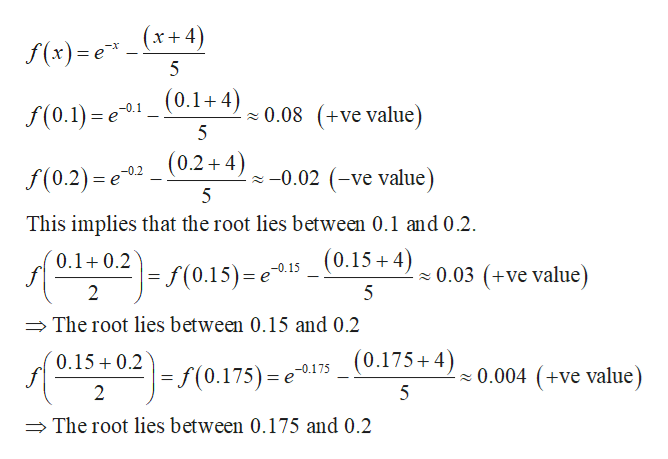# How do you find the roots of the equation f(x)=e-x-(x+4)/5 without a graph?

Question
9 views

How do you find the roots of the equation f(x)=e-x-(x+4)/5 without a graph?

check_circle

star
star
star
star
star
1 Rating
Step 1

Note that intersection of line and exponential curve is a single point which is root of...help_outlineImage Transcriptionclosef(x)= e _(x+4) 5 f(0.1) e01-0.1 0.08 (+ve value) 5 -0.02 (-ve value (0.2)= e02 (0.2+4) f(0.2) 6 22 5 This implies that the root lies between 0.1 and 0.2 (0.15+4) 0.1 0.2 - s(0.15)- e -0.15 0.03 (+ve value) 2 5 The root lies between 0.15 and 0.2 (0.175+4)0.004 (+ve value) 0.15 0. =f(0.175) -0.175 = e 22 2 5 The root lies between 0.175 and 0.2 fullscreen

### Want to see the full answer?

See Solution

#### Want to see this answer and more?

Solutions are written by subject experts who are available 24/7. Questions are typically answered within 1 hour.*

See Solution
*Response times may vary by subject and question.
Tagged in

### Functions